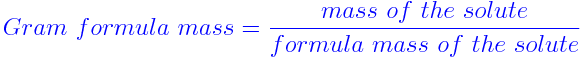# Gram Formula Mass

The gram formula mass of a compound is the amount of that compound that has the same mass in grams as the formula mass in atomic mass unit. An atom of each element has a characteristic mass and in like manner each molecule of a compound has a characteristic formula mass.

When the formula mass of an ionic compound is determined by the addition of its component atomic masses and expressed in grams, it is called the Gram Formula Mass.

Gram Formula Mass is mathematically expressed as## Gram Formula Mass Problems

### Solved Examples

Question 1: Find the gram formula mass of 1mol of KAl(SO4)2.12H2O?

Solution:

1 K = 39
1 Al = 27
2(SO4) = 192 = 2(32 + 16

$$\begin{array}{l}\times\end{array}$$
4)
12 H2O = 216 = 12(2 + 16)

Therefore the gram formula mass is
1 mol = 474g

Question 2: Find the gram formula mass of 1mole of CaCO3?

Solution:

1 Ca = 40
1 C = 12
3 O = 48 = 3

$$\begin{array}{l}\times\end{array}$$
16

Therefore the gram formula mass of CaCO3 is

CaCO3 = 100 formula mass

CaCO3 = 100g = gram formula weight.

#### 1 Comment

1. Havish

your explanation has helped me so much. thank you